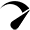# best solar panels for home

## Lithium (LiFePO4) Battery Runtime Calculator

Use our lithium battery runtime (life) calculator to find out how long your lithium (LiFePO4, Lipo, Lithium Iron Phosphate) battery will last running a load. Calculator Assumption How To Use Lithium Battery Runtime Calculator? 1- Enter the battery capacity and select its unit. The unit types are amp-hours (Ah), and Miliamps-hours (mAh). Choose according to your …

## Lead Acid Battery Life Calculator: (SLA, AGM, Gel)

Use our lead-acid battery life calculator to find out how long a Sealed Lead Acid (SLA), AGM, Gel, and Deep cycle lead-acid battery will last running a load. Calculator Assumptions How To Use This Calculator? Step 1: Enter the battery capacity and select the unit type. The unit types are amp-hours (Ah), and milliamp-hours (mAh). Step …

## Lithium Battery Watt Hour Calculator: (mAh / Ah ↔ Wh)

Use our lithium (LiFePO4) battery watt-hour calculator to convert the battery capacity from amp hours (Ah), or milliamp hours (mAh) to watt hours (Wh). How To Use This Calculator? 1 – Enter the battery capacity and select the unit type. The unit types are amp-hours (Ah), and milliamp-hours (mAh). For example, if you have a 100ah …

## Battery Runtime Calculator: How Long Does Battery Last?

How long will your battery last? find out with our easy-to-use battery runtime calculator. Calculator Assumptions Limitations Of This Calculator How To Use Our Battery Runtime Calculator? 1. Enter battery capacity in amp-hours (Ah): If the battery capacity is mentioned in watt-hours (Wh), Divide the watt-hours by battery voltage (V) to find out the battery capacity …

## Solar Panel Size Calculator – Charge Your Battery In Desired Hours

Use our solar panel size calculator to find out what size solar panel you need to charge your battery in desired time. Simply enter the battery specifications, including Ah, volts, and battery type. Also the charge controller type and desired charge time in peak sun hours into our calculator to get your result Calculator Assumptions How To …

## Solar Battery Charge Time Calculator (12v, 24v, 48v)

Use our solar battery charge time calculator to find out how long will it take to charge a battery with solar panels. How To Use Our Solar Battery Charge Time Calculator? To use the calculator, follow these steps: 1. Enter the total solar system size in watts: If you have multiple solar panels connected together, add …

## Solar Panel Amps Calculator (Watts to Amps)

Solar Panel Amps Calculator Use our solar panel amps calculator to calculate the solar panel amps or convert solar panel watts to amps. How To Use This Calculator? Some Key Points Before You Leave We usually measure or convert the watts into amps of solar panels to figure out how much current (amps) is being …

## Battery Amp hours To Watts (Ah to Watts) Calculator

Use our battery amp-hour (Ah) to watts calculator to find out the battery capacity in watt-hours. How To Use This Calculator? How To Calculate Watts In A Battery? Watts is the unit that represents the total number of power. So to calculate watts from Ah use this formula. Watts = Amps*volts Multiplying the value of …

## How to Calculate Solar Panel Battery and Inverter?

The solar calculation for home Step 1:- Home electrical load calculation? Step 2:- Inverter Selection? Step 3:-Battery Selection? Step 4:-Solar Panel Selection? Step 1:- Home electrical load calculation? 4 Led Light    = 4×25 = 100W 2 fan              = 2×80 = 160W 1 Tube Light = 1×40 = 40W 1 Led TV        = 1×50 = 50W Total Load = 350W …

## How to Buy Solar Panel on Loan?

The consumption of electricity in homes has been increasing over the years. It varies from season to season. If a household gets an electricity bill of Rs. 2000 during the rainy season, it can easily go up to Rs. 5000 during hot summer days, which is expensive for a common man. The family also does not want to run …

Scroll to TopOptimized by Seraphinite Accelerator
Turns on site high speed to be attractive for people and search engines.